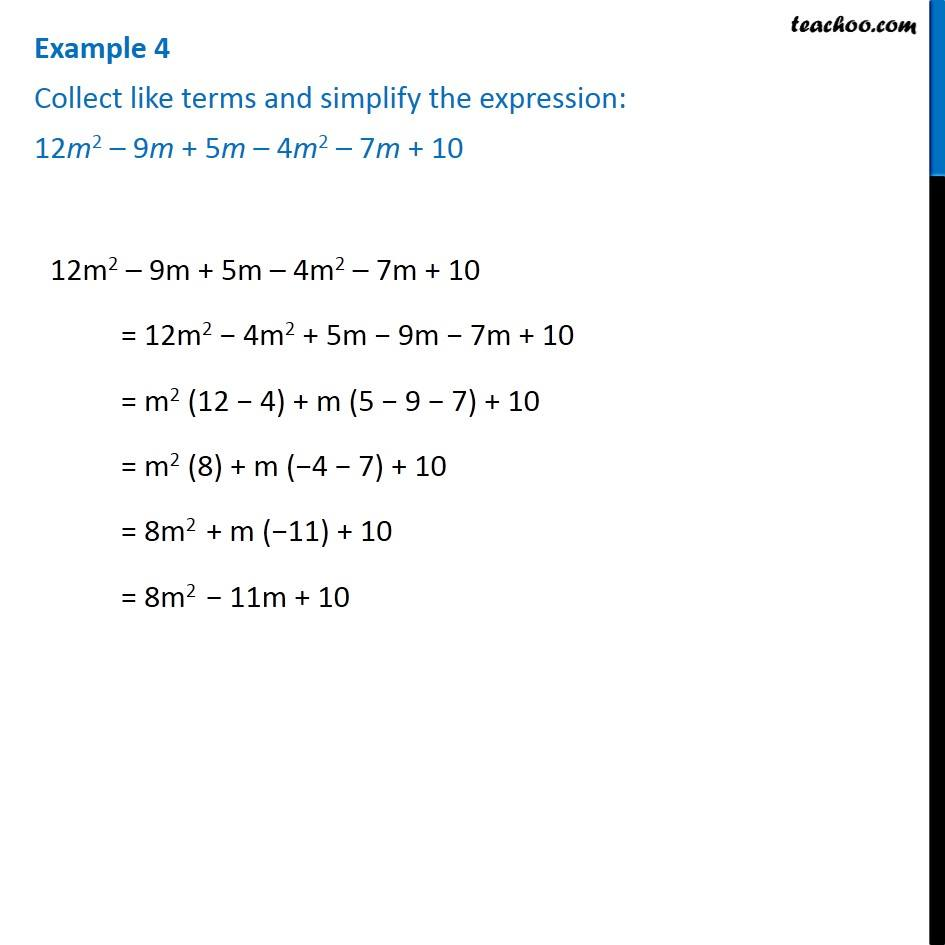Addition and Subtraction of Algebraic Expressions

Chapter 10 Class 7 Algebraic Expressions
Concept wiseLearn in your speed, with individual attention - Teachoo Maths 1-on-1 Class

### Transcript

Question 1 Collect like terms and simplify the expression: 12m2 – 9m + 5m – 4m2 – 7m + 10 12m2 – 9m + 5m – 4m2 – 7m + 10 = 12m2 − 4m2 + 5m − 9m − 7m + 10 = m2 (12 − 4) + m (5 − 9 − 7) + 10 = m2 (8) + m (−4 − 7) + 10 = 8m2 + m (−11) + 10 = 8m2 − 11m + 10